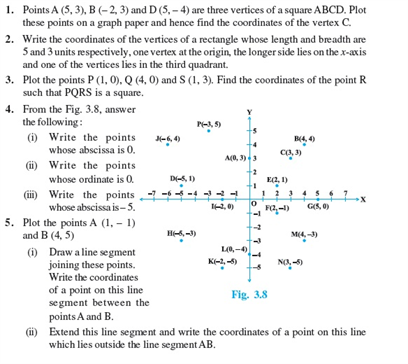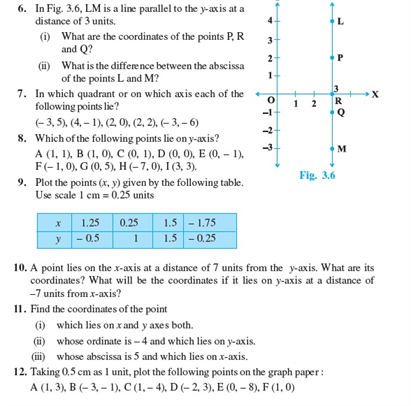# Revision Notes For CBSE Class 9 Math Chapter -3 Coordinate Geometry

A coordinate geometry can be simply defined as a branch of geometry, where the position of the points on the plane is defined with the help of an ordered pair of numbers known as coordinates.

In the coordinate system, a coordinate graph contains a rectangular grid with two coordinate lines called as axes. The horizontal position is represented by X -coordinate and its vertical position is represented by Y- coordinate. They are typically written as an ordered pair (X, Y).

Listed below are some applications or uses of the coordinate geometry.

Coordinate Geometry is mainly used for:

• Calculating the distance between the two given points.
• To describe the equations of different geometric figures.
• Finding the equation, midpoint, or slope of a line segment.
• For calculating the area and the perimeter of a given polygon.
• To conclude the given set of lines are parallel or perpendicular.

Check here for the free CBSE Class 9 Math revision notes for Chapter 3- Coordinate Geometry.